# Analyzing earthquake data¶

This notebook is an element of the risk-engineering.org courseware. It can be distributed under the terms of the Creative Commons Attribution-ShareAlike licence.

Author: Eric Marsden eric.marsden@risk-engineering.org.

This notebook contains an introduction to the use of Python and SciPy to analyze data concerning earthquakes. See the associated course materials for background information and to download this content as a Jupyter notebook.

In :
import numpy
import scipy.stats
import pandas
import matplotlib.pyplot as plt
plt.style.use("bmh")


The USGS provides data on earthquakes that occurred throughout the world at https://earthquake.usgs.gov/earthquakes/search/. For convenience, we have extracted data for 2017 earthquakes of magnitude larger than 5 in CSV format.

In :
data = pandas.read_csv("https://risk-engineering.org/static/data/earthquakes-2017.csv")

Out:
time latitude longitude depth mag magType nst gap dmin rms ... updated place type horizontalError depthError magError magNst status locationSource magSource
0 2017-12-31T20:59:02.500Z -53.0266 -118.3468 10.00 5.1 mb NaN 37.0 30.620 0.85 ... 2018-03-17T01:54:41.040Z Southern East Pacific Rise earthquake 13.7 1.8 0.053 117.0 reviewed us us
1 2017-12-31T20:27:49.390Z -8.1161 68.0644 10.00 5.1 mww NaN 59.0 12.965 0.72 ... 2018-03-17T01:54:41.040Z Chagos Archipelago region earthquake 6.5 1.8 0.062 25.0 reviewed us us
2 2017-12-31T14:53:31.580Z 5.4949 124.9006 30.80 5.1 mww NaN 60.0 1.703 1.01 ... 2018-03-17T01:54:40.040Z 40km S of Daliao, Philippines earthquake 6.7 4.0 0.073 18.0 reviewed us us
3 2017-12-31T14:51:58.200Z -11.8634 165.4973 9.55 5.1 mb NaN 74.0 5.963 1.03 ... 2018-03-17T01:54:40.040Z 132km SSW of Lata, Solomon Islands earthquake 9.1 4.1 0.059 92.0 reviewed us us
4 2017-12-31T03:48:57.420Z 29.6759 129.3045 162.80 5.0 mww NaN 89.0 2.972 0.77 ... 2018-03-17T01:54:40.040Z 146km N of Naze, Japan earthquake 7.6 4.2 0.065 23.0 reviewed us us

5 rows × 22 columns

In :
len(data)

Out:
1559

Let's clean up the data a little.

In :
data.time = pandas.to_datetime(data.time)
data.sort_values("time", inplace=True)


Plot the data to see whether we can identify any visible patterns.

In :
fig = plt.figure()
fig.set_size_inches(20, 4)
plt.plot(data.time, data.mag, ".");
plt.ylabel("Magnitude");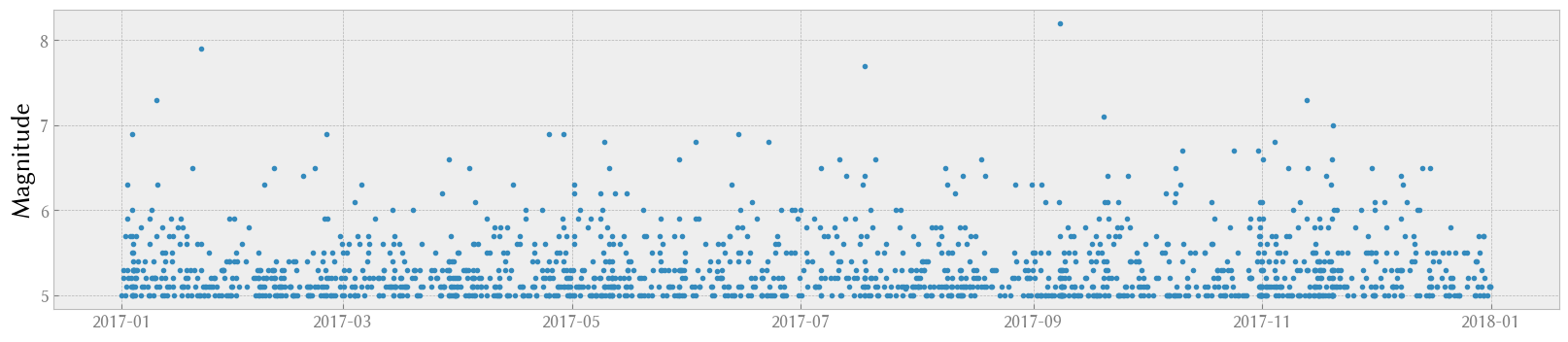There's no visible trend in this time series.

Let's examine the distribution of earthquake magnitudes (recall that we only have data for earthquakes of magnitude larger than 5).

In :
data.mag.hist(density=True, alpha=0.5, bins=30)
plt.xlabel("Earthquake magnitude")
plt.title("Histogram of earthquake magnitudes");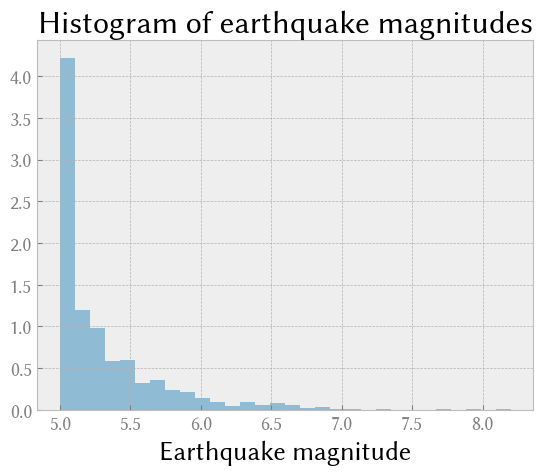Earthquake arrivals follow a Poisson process. This means that interarrival times follow an exponential distribution.

In :
duration = data.time.max() - data.time.min()
density = len(data) / float(duration.days)
density  # events per day

Out:
4.282967032967033

We can attempt to fit an exponential distribution to the interarrival times.

In :
# calculate the time delta between successive rows and convert into days
interarrival = data.time.diff().dropna().apply(lambda x: x / numpy.timedelta64(1, "D"))
support = numpy.linspace(interarrival.min(), interarrival.max(), 100)
interarrival.hist(density=True, alpha=0.5, bins=30)
plt.plot(support, scipy.stats.expon(scale=1/density).pdf(support), lw=2)
plt.title("Duration between 5+ earthquakes")
plt.xlabel("Days");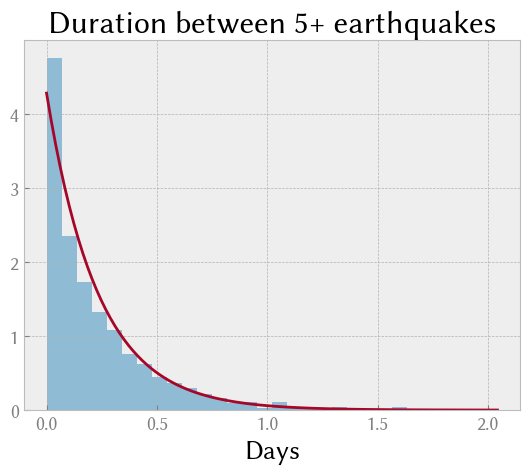It looks like the inter-event times do indeed fit an exponential distribution well. To check, generate a probability plot against the exponential distribution. This shows quantiles from our sample against quantiles from the theoretical distribution. If the points are close to the diagonal red line, the sample closely follows the distribution.

In :
shape, loc = scipy.stats.expon.fit(interarrival)
scipy.stats.probplot(interarrival,
dist="expon", sparams=(shape, loc),
plt.title("Exponential probability plot of interarrival time");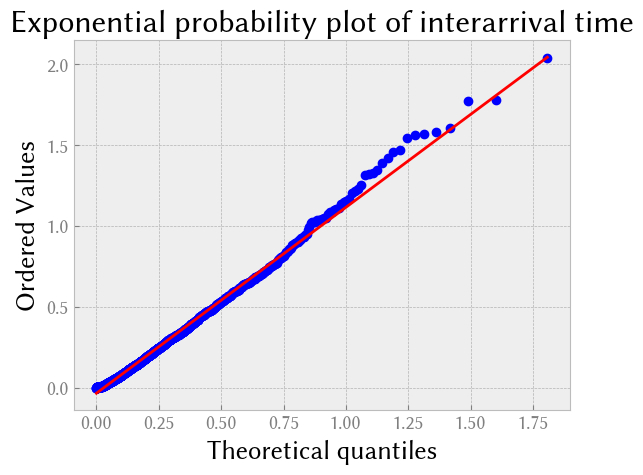A correlogram or autocorrelation plot tests whether elements of a time series are positively correlated, negatively correlated, or independent of each other. This is important to detect trends or cycles in time series data.

In :
plt.acorr(interarrival, maxlags=200)
plt.title("Autocorrelation of earthquake timeseries data");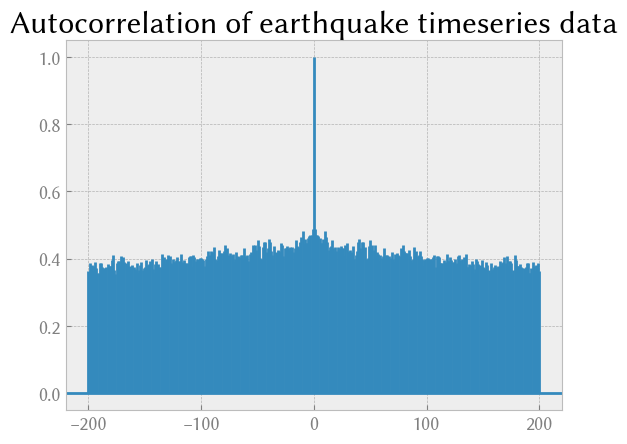There is no visible trend in this time series data (note that we are only examining large earthquakes; the same plot concerning all earthquakes would likely show correlations at small numbers of days, because large earthquakes tend to trigger small aftershocks in the following days, or sometimes large quakes are preceded by small earthquakes).

In [ ]: